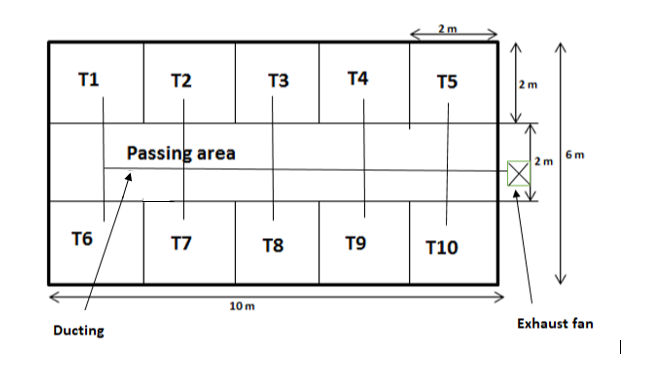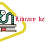# Exhaust fan selection/Bathroom fan selection

## Exhaust fan selection/Bathroom fan selection

We have to find two things for fan selection

1)Air flow(CFM)

2)Static Pressure(wg)

Calculation:(This is a rough drawing )

1)Air flow:

As per ASHARE, 1 public continuous toilet CFM=50 CFM

10 public continuous toilet CFM=10*50=500 CFM

Passing area=10 m*2 m=20 m2=20*10.76=215.2 sq ft

As per ASHARE, For passing area 1 square feet = 1 CFM

Hence Passing area =215.2sq ft=215.2 CFM

Total Exhaust CFM=500+215.2=715.2 CFM

Fresh air cfm=715.2*0.8=572.16 CFM =572.16*1.7=972.6 CMH

If you know more about CFM calculation, then go to below link

https://librarykeeda.blogspot.com/2020/06/toilet-ventilation.html

2)Static Pressure:

• It is one of the most important factors in HVAC design.
• It refers to the resistance to airflow in a heating and cooling system’s components and duct work.
• Heat loss in 100 ft per 0.08 wg (means in 100 ft duct statis loss 0.08” wg)
• For 1 m of straight duct static pressure loss 0.004” wg.
• For 1, 90o elbow static pressure loss 0.2” of wg.
• For 1, 45o elbow static pressure loss 0.1” of wg.

Calculation:

From above drawing select the ducting layout;

After this we have to choose longest route of this drawing

• Total duct length(straight piece)=10+3=13m
• For 1 m of straight duct static pressure loss 0.004” wg
• Hence, For 13 m of straight duct static pressure loss =0.004*13=0.052 wg
• As per above drawing one elbow is there
• Hence For 1, 90o elbow static pressure loss 0.2” of wg
• Total Static pressure=0.052+0.2=0.252” of wg

1.1.2.